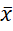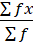### Measures of Central Tendency for Grouped Data

Mathematics SSS 2 Third Term

WEEK 8

Measures of Central Tendency for Grouped Data

Performance Objectives

Students should be able to;

1. Determine the Mean of grouped data
2. Determine the Median of grouped data
3. Determine the Mode of grouped data

Mean of Grouped Data

Content

Mean for grouped data can be calculated in two ways;

(i) Mean for problems without assumed mean

x= fxfWhere x is the class mark or class midpoint

(ii) Mean of problems with assumed mean

View More